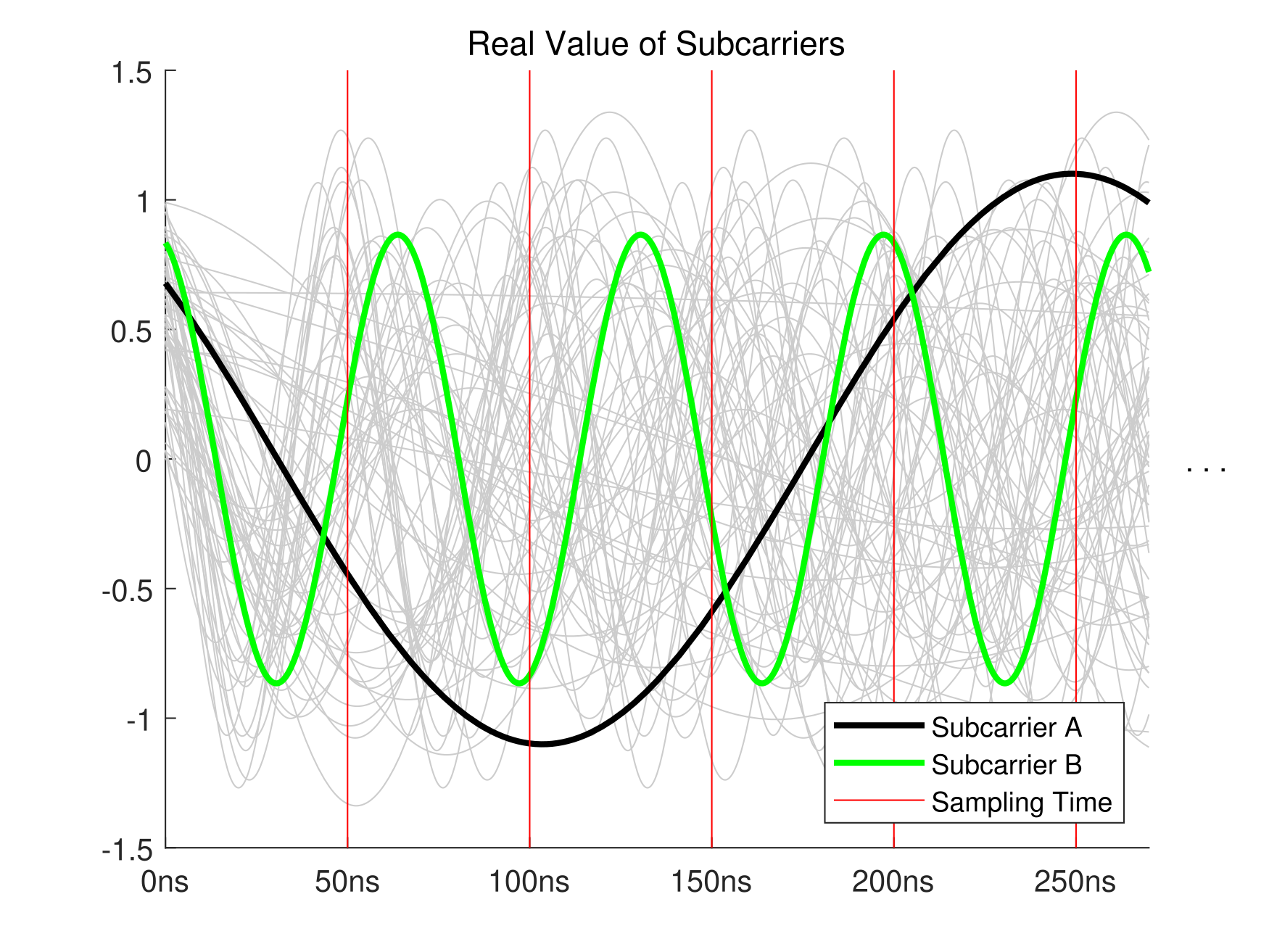블로그
글 개요
2020년 02월 04일 20:25무선 네트워크/기본 개념댓글 수0개

# Where is the orthogonality in OFDM?

태그
본문

## Mathematical Concept of "Orthogonality"

Generally orthogonality means that the inner product of two vector is zero.

There's not only a concept of the inner product of vectors, but the inner product of functions, and this can be expressed as a definite integral.

$$\left\lbrace \begin{array}{ccc}<f,g>=\int_a^b f(x)g(x)~dx & & \textrm{Inner}\;\textrm{Prod.}\;\textrm{of}\;\textrm{Real}\;\textrm{Fn.}\\<f,g>=\int_a^b f(x)\overline{g(x)} ~dx & & \textrm{Inner}\;\textrm{Prod.}\;\textrm{of}\;\textrm{Complex}\;\textrm{Fn.}\end{array}\right.$$

Therefore, we can say that two functions are orthogonal if their inner product value is zero and this can be applied to complex signals.

## Orthogonality of OFDM Subcarriers

### Mathematical Expression of OFDM Signal

The OFDM signal can be expressed as below.

$$\left\lbrace \begin{array}{ccc}S(t)=\frac{1}{N}\sum_{n=0}^{N-1} A_n (t)e^{j\left\lbrack \omega_n t+\phi_n (t)\right\rbrack } & & \textrm{OFDM}\;\textrm{Signal}\\S_n (t)=A_n (t)e^{j\left\lbrack \omega_n t+\phi_n (t)\right\rbrack } & & \textrm{Signal}\;\textrm{of}\;\textrm{Each}\;\textrm{Subcarrier}\end{array}\right.$$

$N$ is the number of subcarriers, $A_n$ is amplitude of each subcarrier, and $\phi_n$ is phase of them.

$A_n (t)$ and $\phi_n (t)$ has a constant value during a symbol duration and $\omega_n =\omega_0 +n\Delta \omega$.

Suppose that the sampling rate is $1/T_s$, then a period of one OFDM symbol is $T=NT_s$ because we should sample $N$ times in one symbol. Also suppose that $\omega_0 =0$, then $\omega_n$ becomes $n\Delta \omega$.

We can now write a simple sampled signal expression: $S(tT_s )=\frac{1}{N}\sum_{n=0}^{N-1} \textrm{C}e^{jn\Delta \omega tT_s } ~~(0\le t<T)$

$\textrm{C}$ is a const from amplitude and phase values at a specific symbol.

The OFDM signal is generated from IDFT calculation, so let's compare above with general IDFT expression.

$$\left\lbrace \begin{array}{ccc}S(tT_s )=\frac{1}{N}\sum_{n=0}^{N-1} \textrm{C}e^{jn\Delta \omega tT_s } & & \textrm{Simplified}\;\textrm{OFDM}\;\textrm{Signal}\\x(tT_s )=\frac{1}{N}\sum_{n=0}^{N-1} X(tT_s )e^{j2\pi tn/N} & & \textrm{General}\;\textrm{IDFT}\;\textrm{Expression}\end{array}\right.$$

So, $\Delta \omega =\frac{2\pi }{T}$. This is a condition for the orthogonality. Why?

### Dealing with Orthogonality

Let's try to show that subcarrier $\alpha$ and $\beta$ is orthogonal. We should solve definite integral of two signal of each subcarrier.

$$\int_0^T S_{\alpha } (t)\overline{S_{\beta } (t)} ~dt$$

For convinience of thinking, the amplitude part and phase part can be expressed as a symbol $C$ because they are constant. Also suppose that the lowest frequency is 0 ($\omega_0 =0$).

$$C\int_0^T e^{j(\alpha \Delta \omega )t} e^{-j(\beta \Delta \omega )t} ~dt=C\int_0^T e^{jt\Delta \omega (\alpha -\beta )} ~dt$$

Then the trigonometric form of the integration is $C\int_0^T \cos (t\Delta \omega k)+j\sin (t\Delta \omega k)~dt$ if $k=\alpha -\beta$.

This equals to $C\int_0^T \cos (\frac{2\pi tk}{T})+j\sin (\frac{2\pi tk}{T})~dt$ and $k$ is an integer!

When we solve the integral: $C(0+0j)=0$

So, for any subcarrier $\mathbf{\alpha}$ and $\mathbf{\beta}$, they are orthogonal!

## MATLAB Example

% Select Two Subcarriers
sub_a = 11; % Subcarrier A [1 64]
sub_b = 48; % Subcarrier B [1 64]

% Environment Configuration
symbol_duration = 3.2e-6; % 3.2 microseconds
rx_sampling_time = 5e-8:5e-8:symbol_duration; % 20MHz
N = 64; % 64 subcarriers

% One Symbol Signal Generation
rng('shuffle');
syms t;
% oscillator_resolution = (symbol_duration / (N * 1e+2));
signal = sym(zeros(N));
for i = 1:N
signal(i) = (rand(1) + rand(1) * 1i) * ...
exp(1i * i * 2 * pi / symbol_duration * t);
end

% Plot Signals
figure(1); clf;
hold on;
plot(NaN, 'Color', [0 0 0], 'LineWidth', 2);
plot(NaN, 'Color', 'g', 'LineWidth', 2);
plot(NaN, 'Color', 'r');
for i = 1:N
if i ~= sub_a && i ~= sub_b
fplot(real(signal(i)), [0 27e-8], 'Color', [0 0 0] + 0.8);
end
end
fplot(real(signal(sub_a)), [0 27e-8], 'Color', [0 0 0], 'LineWidth', 2);
fplot(real(signal(sub_b)), [0 27e-8], 'Color', 'g', 'LineWidth', 2);
for i = 1:5
plot([i * 5e-8 i * 5e-8], [-1.5 1.5], 'Color', 'r');
end
hold off;
ylim([-1.5 1.5]);
xlim([0 27e-8]);
xticks(0:5e-8:25e-8);
xticklabels({'0ns' '50ns' '100ns' '150ns' '200ns' '250ns'});
text(28e-8, 0, '. . .');
legend('Subcarrier A', 'Subcarrier B', 'Sampling Time', 'Location', 'southeast');
title('Real Value of Subcarriers');

% Inner Product
signal_a = signal(sub_a);
signal_b_conj = conj(signal(sub_b));
product = int(signal_a * signal_b_conj, t, 0, symbol_duration);

% Display Product Result
figure(2); clf;
scatter(real(double(product)), imag(double(product)), 1200, 'Marker', '*', ...
'MarkerEdgeColor', [1 0.8 0.4] - 0.1, 'LineWidth', 2);
ylim([-1e-15 1e-15]);
yticks(-0.8e-15:0.2e-15:0.8e-15);
ylabel('Imag');
xlim([-1e-15 1e-15]);
xticks(-0.8e-15:0.2e-15:0.8e-15);
xlabel('Real');
pbaspect([1 1 1]);
ax = gca;
ax.XAxisLocation = 'origin';
ax.YAxisLocation = 'origin';
text(1.2e-16, 1.2e-16, '↙ Zero?');
title({'Product Result =', strtrim(evalc('disp(double(product))'))});(Left) Figure 1 / (Right) Figure 2

가장 위로